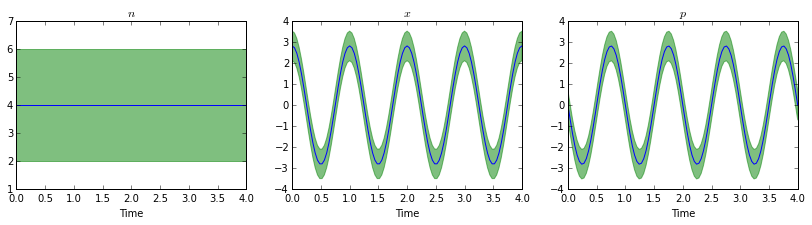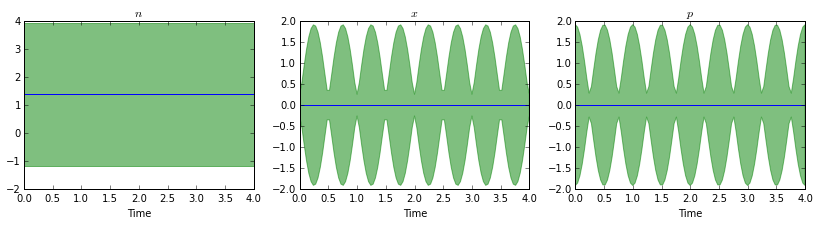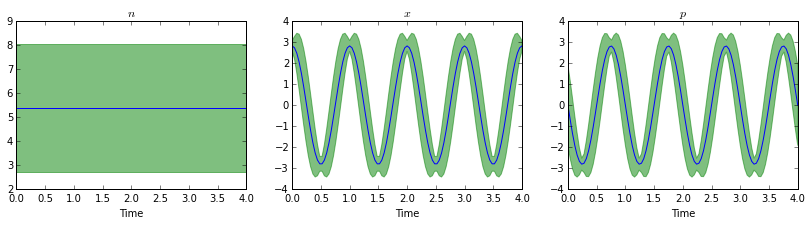# Lecture 9: Squeezed states of a quantum harmonic oscillator¶

Author: J.R. Johansson, [email protected]

http://dml.riken.jp/~rob/

Latest version of this ipython notebook lecture is available at: http://github.com/jrjohansson/qutip-lectures

In :
%matplotlib inline
import matplotlib.pyplot as plt
import numpy as np

In :
from IPython.display import HTML
from matplotlib import animation

In :
from qutip import *


## Introduction¶

In quantum mechanics, each measurement of an observable (corresponding to an Hermitian operator) results in stochastic outcome that follows some probability distribution. The expectation value of the operator is the average of many measurement outcomes, and the variance of the operator describes the uncertainty in the outcomes.

This uncertainty is intrinsic in quantum mechanics, and cannot be eliminated. The Heisenberg uncertainty principle describes the minumum uncertainly for pairs of noncommuting operators. For example, the operators such $x$ and $p$, which satisfy the commutation relation $[x, p] = i\hbar$, must always satisfy $(\Delta x)^2 (\Delta p)^2 > \hbar/2$ .

A state that satisfy

$(\Delta x)^2 (\Delta p)^2 = \hbar/2$

is called a minimum uncertainty state, and a state for which for example

$(\Delta x)^2 < \hbar/2$

is called a squeezed state. Note that in this case $(\Delta p)^2$ must be larger than $\hbar/2(\Delta x)^2$ for the Heisenberg relation to hold. Squeezing a quantum state so that the variance of one operator $x$ is reduced below the minimum uncertainty limit therefore necessarily amplify the variance of operators that do not commute with $x$, such as $p$.

For harmonic modes, squeezing of $x$ or $p$ is called quadrature squeezing, and it is probably the most common form of squeezing.

In this QuTiP notebook we look at how expectation values and variances of the quadrature operators $x$ or $p$ of a single harmonic mode evolve in time when initially in different kinds of squeezed states.

## Parameters¶

In :
N = 35
w = 1 * 2 * np.pi              # oscillator frequency
tlist = np.linspace(0, 4, 101) # periods

In :
# operators
a = destroy(N)
n = num(N)
x = (a + a.dag())/np.sqrt(2)
p = -1j * (a - a.dag())/np.sqrt(2)

In :
# the quantum harmonic oscillator Hamiltonian
H = w * a.dag() * a

In :
c_ops = []

# uncomment to see how things change when disspation is included
# c_ops = [np.sqrt(0.25) * a]


## Functions for plotting¶

Since we want to repeat the same kind of calculation and visualization for several different states, we first define a few functions that we can reuse for each state later on.

In :
def plot_expect_with_variance(N, op_list, op_title, states):
"""
Plot the expectation value of an operator (list of operators)
with an envelope that describes the operators variance.
"""

fig, axes = plt.subplots(1, len(op_list), figsize=(14,3))

for idx, op in enumerate(op_list):

e_op = expect(op, states)
v_op = variance(op, states)

axes[idx].fill_between(tlist, e_op - np.sqrt(v_op), e_op + np.sqrt(v_op), color="green", alpha=0.5);
axes[idx].plot(tlist, e_op, label="expectation")
axes[idx].set_xlabel('Time')
axes[idx].set_title(op_title[idx])

return fig, axes

In :
from base64 import b64encode

def display_embedded_video(filename):
video = open(filename, "rb").read()
video_encoded = b64encode(video).decode("ascii")
video_tag = '<video controls alt="test" src="data:video/x-m4v;base64,{0}">'.format(video_encoded)
return HTML(video_tag)


## Coherent state¶

For reference, let's first look at the time evolution of a coherent state.

In :
psi0 = coherent(N, 2.0)

In :
result = mesolve(H, psi0, tlist, c_ops, [])

In :
plot_expect_with_variance(N, [n, x, p], [r'$n$', r'$x$', r'$p$'], result.states);In :
fig, axes = plt.subplots(1, 2, figsize=(10,5))

def update(n):
axes.cla()
plot_wigner_fock_distribution(result.states[n], fig=fig, axes=axes)

anim = animation.FuncAnimation(fig, update, frames=len(result.states), blit=True)

anim.save('/tmp/animation-coherent-state.mp4', fps=20, writer="avconv", codec="libx264")

plt.close(fig)

In :
display_embedded_video("/tmp/animation-coherent-state.mp4")

Out:

## Squeezed vacuum¶

In :
psi0 = squeeze(N, 1.0) * basis(N, 0)

In :
result = mesolve(H, psi0, tlist, c_ops, [])

In :
plot_expect_with_variance(N, [n, x, p], [r'$n$', r'$x$', r'$p$'], result.states);In :
fig, axes = plt.subplots(1, 2, figsize=(10,5))

def update(n):
axes.cla()
plot_wigner_fock_distribution(result.states[n], fig=fig, axes=axes)

anim = animation.FuncAnimation(fig, update, frames=len(result.states), blit=True)

anim.save('/tmp/animation-squeezed-vacuum.mp4', fps=20, writer="avconv", codec="libx264")

plt.close(fig)

In :
display_embedded_video("/tmp/animation-squeezed-vacuum.mp4")

Out:

## Squeezed coherent state¶

In :
psi0 = displace(N, 2) * squeeze(N, 1.0) * basis(N, 0)  # first squeeze vacuum and then displace

In :
result = mesolve(H, psi0, tlist, c_ops, [])

In :
plot_expect_with_variance(N, [n, x, p], [r'$n$', r'$x$', r'$p$'], result.states);In :
fig, axes = plt.subplots(1, 2, figsize=(10,5))

def update(n):
axes.cla()
plot_wigner_fock_distribution(result.states[n], fig=fig, axes=axes)

anim = animation.FuncAnimation(fig, update, frames=len(result.states), blit=True)

anim.save('/tmp/animation-squeezed-coherent-state.mp4', fps=20, writer="avconv", codec="libx264")

plt.close(fig)

In :
display_embedded_video("/tmp/animation-squeezed-coherent-state.mp4")

Out:

### Software versions¶

In :
from qutip.ipynbtools import version_table; version_table()

Out:
SoftwareVersion
IPython2.3.0
Cython0.20.1
OSposix [darwin]
matplotlib1.4.2
Python3.4.2 (default, Oct 19 2014, 08:26:16) [GCC 4.2.1 Compatible Apple LLVM 6.0 (clang-600.0.51)]
SciPy0.14.0
QuTiP3.1.0
Numpy1.9.1
Tue Jan 20 16:53:50 2015 JST